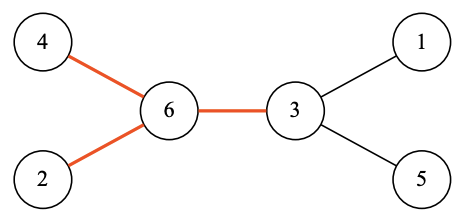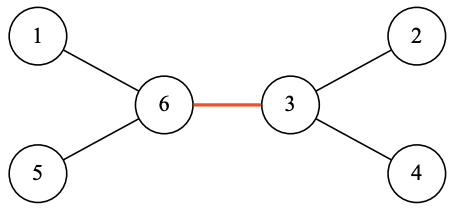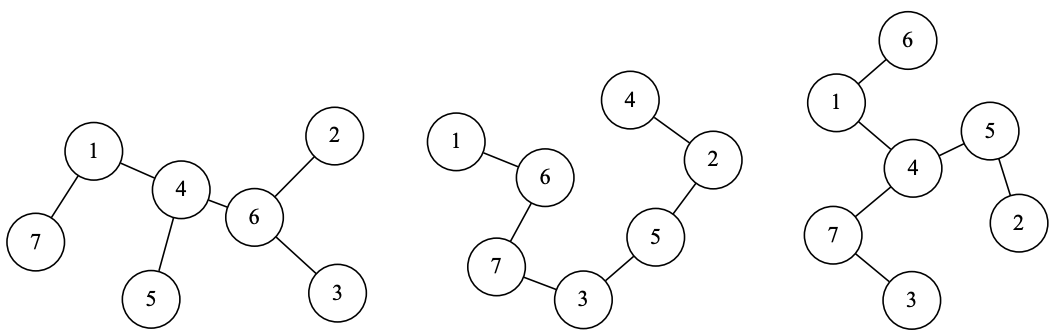시간 제한메모리 제한제출정답맞힌 사람정답 비율
10 초 512 MB35302382.143%

## 문제

A tree is a connected undirected graph that has no cycles. Consider a tree with n vertices, labeled with integers 1, 2, ..., n. Let’s call an edge (u, v) bad if there is an integer d > 1 such that the label of u and the label of v are both divisible by d. For example, in the tree below there are three bad edges: (6, 4) are both divisible by 2, (2, 6) are both divisible by 2, and (3, 6) are both divisible by 3:Your goal is to relabel vertices in such a way that the number of bad edges is as small as possible. For example, if you relabel vertices of the tree shown above in the following way, there will be only one bad edge (3, 6):The less bad edges your tree will have the more points you will get.

This is an output-only problem. You need to run your program locally and only submit the answer file for each input file.

## 입력

Each input file contains several test cases.

The first line of the input file contains the number of test cases in this input file.

The first line of test case description contains a single integer n, the number of the vertices in the tree.

Each of the following n − 1 lines contains two integers u and v (1 ≤ u, v ≤ n), vertices connected by the edge.

All trees in a single file have the same number of vertices.

## 출력

For each test case print one line containing exactly n different integers from 1 to n — labels assigned to vertices 1, 2, . . . , n.

## 점수

For each input file, let the total number of edges in all test cases of this input file be M, the total number of bad edges in your solution be X, and R =X/M . Your score for the input file is calculated as following:

• if R > 0.4, your score will be 0;
• if 0.33 < R ≤ 0.4, your score will be 1;
• if 0.26 < R ≤ 0.33, your score will be 2;
• if 0.19 < R ≤ 0.26, your score will be 3;
• if 0.12 < R ≤ 0.19, your score will be 4;
• if 0.05 < R ≤ 0.12, your score will be 5;
• if 0.01 < R ≤ 0.05, your score will be 6;
• if 0.005 < R ≤ 0.01, your score will be 7;
• if 0.001 < R ≤ 0.005, your score will be 8;
• if 0 < R ≤ 0.001, your score will be 9;
• if R = 0, your score will be 10.

## 예제 입력 1

2
6
1 3
3 5
3 6
6 4
6 2
6
1 2
1 3
1 4
1 5
1 6


## 예제 출력 1

2 5 3 1 4 6
5 1 2 3 4 6


## 힌트

First test case is shown in the problem statement above. There is one bad edge (6, 3) after relabeling, because both 6 and 3 are divisible by 3.

In the second test case there will be edges (5, 1), (5, 2), (5, 3), (5, 4) and (5, 6). None of them are bad.

There are 10 edges in the input file and 1 bad edge in the answer. Thus, M = 10, X = 1, R = 0.1. According to the scoring table, this answer would get 5 points.

Tests have the following structure:

• Input file 1 contains three trees on 7 vertices, depicted below from the left to the right:• Input files 2 and 3 contain 100 random trees on 10 and 30 vertices respectively.
• Input files 4 to 8 contain various randomly generated trees with some special structure (e.g. trees with many leaves, binary trees etc). Distribution of different kinds of trees is roughly the same for
all inputs.
• Input files 9 and 10 contain randomly generated trees of 50 000 and 100 000 vertices respectively.

Initially, label of vertices of all trees in all input files are random.

압축 파일data-2.in으로 채점한다.

## 채점 및 기타 정보

• 예제는 채점하지 않는다.